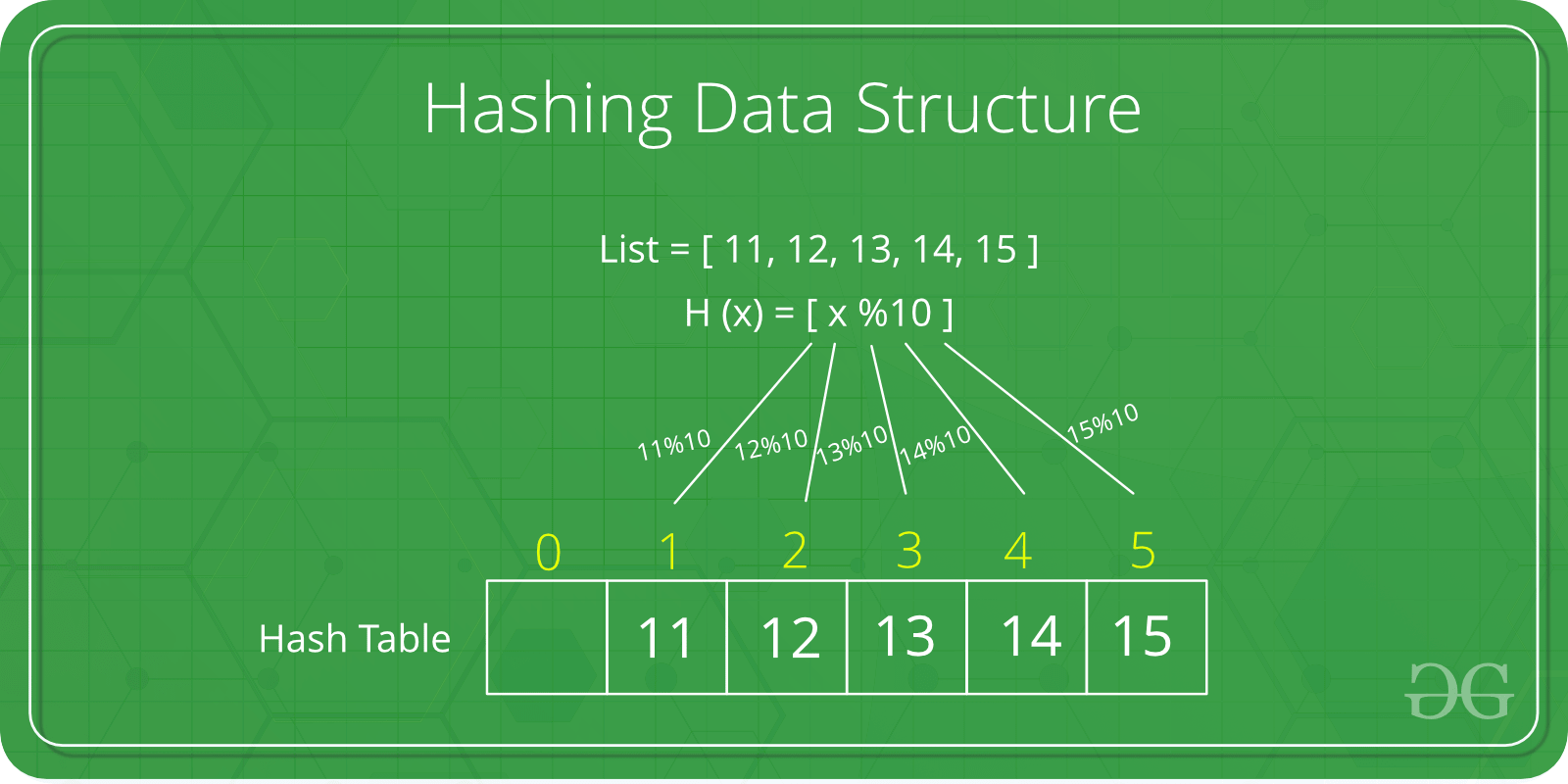# Python 中的哈希表

• 2023-04-24
浙江
• 本文字数：1183 字

阅读完需：约 4 分钟Python 中提供了字典(dict)类型来实现哈希表。字典是一种包含键值对的可变集合，支持常数时间的插入、查找、和删除操作。

hash_table = {}# Inserthash_table['apple'] = 1hash_table['banana'] = 2hash_table['cherry'] = 3# Lookupprint(hash_table['apple'])  # 1print(hash_table['banana'])  # 2print(hash_table['cherry'])  # 3# Deletedel hash_table['banana']print(hash_table)  # {'apple': 1, 'cherry': 3}

hash_table = [None] * 10  # 初始大小为10的哈希表，初始值为Nonedef hash_function(key):    return hash(key) % len(hash_table)  # 使用Python内置哈希函数，对哈希表大小进行取模# Insertkey = 'apple'value = 1index = hash_function(key)hash_table[index] = value# Lookupkey = 'apple'index = hash_function(key)print(hash_table[index])  # 1# Deletekey = 'apple'index = hash_function(key)hash_table[index] = None## 评论